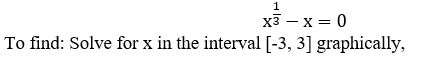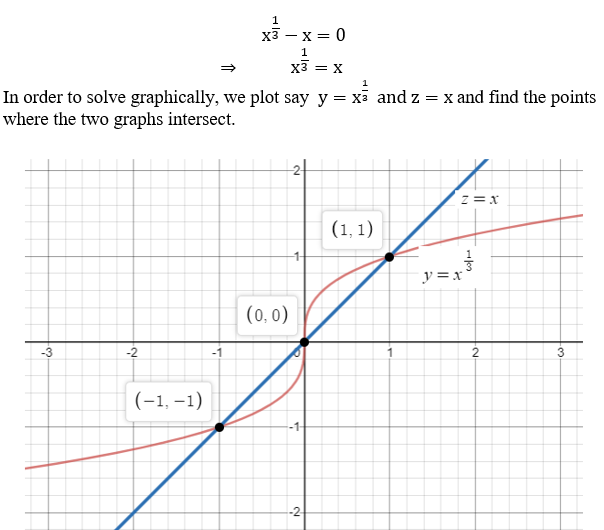# Solve the equation graphically in the given interval. State each answer rounded to two decimals. (Enter your answers as a comma-separated list.)x1/3 − x = 0;   [−3, 3]x =  ( the x=1 is not correct)

Question
1 views

Solve the equation graphically in the given interval. State each answer rounded to two decimals. (Enter your answers as a comma-separated list.)

x1/3 − x = 0;   [−3, 3]
x =

( the x=1 is not correct)
check_circle

Given an equationBegin with the given equation,...

### Want to see the full answer?

See Solution

#### Want to see this answer and more?

Solutions are written by subject experts who are available 24/7. Questions are typically answered within 1 hour.*

See Solution
*Response times may vary by subject and question.
Tagged in